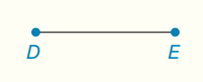Chapter 7.CT, Problem 3CTElementary Geometry For College St...

7th Edition
Alexander + 2 others
ISBN: 9781337614085

Solutions

Chapter
SectionElementary Geometry For College St...

7th Edition
Alexander + 2 others
ISBN: 9781337614085
Textbook Problem

Draw and describe the locus of points in the plane that are equidistant from the endpoints of D E ¯ .To determine

To draw and describe:

The locus of points in the plane that are equidistant from the endpoints of DE¯.

Explanation

Definition:

Locus of points that are equidistant from the endpoints of a line segment.

For example:

The perpendicular bisector l of RS¯.

Calculation:

A theorem in section one of this chapter states that the locus of the two end points of a line segment is the perpendicular bisector of that line segment

Still sussing out bartleby?

Check out a sample textbook solution.

See a sample solution

The Solution to Your Study Problems

Bartleby provides explanations to thousands of textbook problems written by our experts, many with advanced degrees!

Get Started

Find more solutions based on key concepts